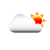## Learning Python: How to write FOR loop in Python

When one transitions from one programming language to a new one, there are always questions about how do I do something in this new language that I am used to doing in current language. In this post, I will answer the question about how to write for loop in Python.

I will use following code snippet to talk about this topic. For a tutorial, I wrote some code to show how to calculate coordinates based on angular rotation.

```from math import pi,sin,cos

rotation = 6.0
for angle in range(1,60):
angleDegree = rotation * angle
angleRadians = angleDegree * (pi / 180.0)
print(f"{x_new}: {y_new}")
```

## Syntax

Purpose of for loop is to iterate over each element in the collection that have been specified after in. Each element in that collection is returned in variable name specified after for. In my example, the collection is created by using range function.

## Start Index and Step

In most languages, you get to specify increment step in for loop. In Python, if you need to specify increment step, then you will need to create the range accordingly. E.g if you want every second index in that range, then use following code to create range.

```for angle in range(1,60,2):
```

You can specify starting index of for loop in range function itself. E.g, if I want to start the loop from ZERO index, then use the following code.

```for angle in range(0,60,2):
```

## Weather

#### 24.2 °C / 75.6 °FClouds